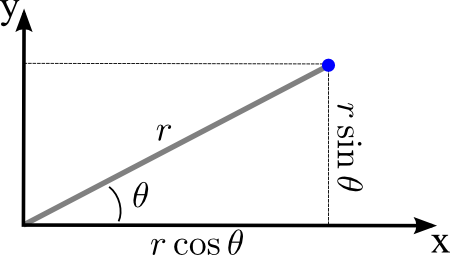# Math Insight

### Image: Polar coordinates to Cartesian coordinatesTo calculate the Cartesian coordinates of a point with polar coordinates $(r,\theta)$, form a right triangle formed by the line segment from the origin to the point and the projection of this line segment on the $x$-axis. The $x$-component is determined by the leg of the triangle adjacent to the angle $\theta$, so $x=r\cos\theta$. The $y$-component is determined by the other leg, so $y=r\sin\theta$.

Image file: polar_coordinates_cartesian.png

Source image file: polar_coordinates_cartesian.svg
Source image type: Inkscape SVG